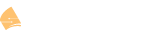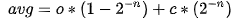# WRED (Weighted Random Early Detection)

This topic is to discuss the following lesson:

Hello Rene,

Thank you very much for the lesson.

but I’m still a little bit confused, Please, correct me if I’m wrong:
based on the command below if it’s set AF probability will be considered:
`random-detect dscp-based`

now if we have AF21 and AF33 the class different but the probability of dropping packet from AF33 more than AF21, correct?
what about if the packets AF21 and AF31? what about if we have AF21 and EF and CS3 and CS4?

also what is the meaning for fair-queue command? what is the impact when you are using it in the policy map?

Thank you,
Samer Abbas

Adding I’m looking for the drop probability for these Marking if they are in the same Policy Map with `random-detect dscp-based`

Thanks,
Samer

Hello Samer

Class 4 has the highest priority, so if you have AF33, it will have a lower drop probability than AF21 for example. But within the same class, the higher the number the higher the drop probability, so AF13 will more likely be dropped compared to AF11. So yes, you are correct.

AF31 is in a higher priority class than AF21, so AF 21 has the higher drop probability.

EF are also considered part of the DSCP based WREN procedure and are given an even higher priority than the AF markings. As for CS3 and CS4, they have a higher drop probability than the AF series of values.

Ultimately, when using the `random-detect dscp-based` command, you are telling the device to use the six bit DSCP value as the criteria for random drops. As Cisco documentation states, the “dscp-based argument enables WRED to use the DSCP value of a packet when calculating drop probability.” This means that the whole value (6 bits) is used, which means that CS, AF and EF values are considered in this calculation.

You are also able to optionally specify the minimum and maximum packet thresholds for the DSCP value using the `random-detect dscp` command.

https://www.cisco.com/c/en/us/td/docs/ios-xml/ios/qos_conavd/configuration/12-4t/qos-conavd-12-4t-book/qos-conavd-oview.html#GUID-654C88AF-0E34-4864-8526-93C6C3615F51

The `fair-queue` command is used to implement Distributed WRED (DWRED). It’s a feature only available in the 7000 series routers. This specific command specifies the number of queues to be reserved for the specific traffic class. You can find out more about DWRED here:
https://www.cisco.com/c/en/us/td/docs/ios-xml/ios/qos_conavd/configuration/15-mt/qos-conavd-15-mt-book/qos-conavd-oview.html#GUID-E89670B1-9EFA-4DDD-8862-58818247DD94

I hope this has been helpful!

Laz

Hi There,

can explain me the average size calculation in WRED. Is queue size calculated based on the bandwidth available to the specific queue or total available bandwidth of link ?

Regards,
Ranganna

Hello Ranganna

When WRED calculates the average queue size, it does so by calculating the actual size of the real queue. Specifically, the average is calculated periodically every few milliseconds. It uses the following formula:• o is the old average calculated the previous time
• n is the weight factor you configure
• c is the current queue size

The maximum size of the physical queue will depend on what kind of interface we’re talking about and what platform it is functioning on.

I hope this has been helpful!

Laz

Hello,

Perhaps a note would be useful informing that the instantaneous queue depth is used for the tail drop (Exponential Weighting Constant chapter).

Also in the formula for the average queue depth:
the (instantaneous_old_average) should be change into (instantaneous - old_average)

Many thanks,
Stefanita

Thanks Stefanita. I fixed this and added something about the instantaneous queue depth.

Rene

1 Like